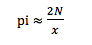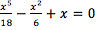Associate Professor of Mathematics Daniela De Silva teaches calculus and analysis and focuses most of her research on partial differential equations. Within this frame, the regularity theory for free boundary/phase transitions problems (for example, determining the changing shape of a melting solid, the most efficient way to insulate an object, flame propagation, and problems from financial mathematics) is what Prof. De Silva finds most interesting; she has published more than a dozen scholarly articles on the topic. She also organizes weekly “Geometry and Analysis” seminars at Columbia University throughout the semester. For this installment, and right in time for Pi Day (March 14), the 2016 Association for Women in Mathematics-Sadosky Research Prize in Analysis winner explains what pi really means. Einstein would be proud (the world celebrates his 138th birthday on Pi Day)!

###Can we start with a quick math lesson? What is pi and why is it seemingly an endless number?

The number pi represents the ratio of a circle's circumference to its diameter. This ratio is constant, regardless of the circle's size. The name pi is in fact derived from the first letter of the Greek word “perimeter.”

The never-ending nature of pi is due to the fact that it is an irrational number (irrational numbers are those that can be written as a decimal not a fraction because of their non-repeating, endless digits). Such numbers cannot be expressed as the ratio of two integers, so no matter how many copies of pi one adds together the answer will never be a whole number. For this reason, the decimal representation of pi contains an infinite number of digits that does not settle into an infinitely repeating pattern. This infinite sequence of numbers is indeed “statistically random,” that is, it compares to the outcome of infinitely many dice rolls. Curiously, it does contain some sequences of digits that may appear non-random, such as a sequence of six consecutive 9’s that begins at the 762nd decimal digit.

### Why is pi so important to mathematicians?

Clearly, pi appears in many formulas from the fields of geometry, particularly those concerning circles, spheres, or ellipses. However, pi is relevant also in situations having apparently nothing to do with geometry! To give an idea of the diverse faces of pi, let me describe one of the many ways in which one can approximate it. This is known as Buffon's needle paradox.

Imagine a common hardwood floor made of parallel strips of wood, each of the same width 1. If we drop a needle of length 1 onto the floor N times and x of those times the needle comes to rest crossing a line, then we can approximate pi as:Surprised?

Beautiful formulas connect the number pi with prime numbers as well: what is the probability that two randomly chosen integers have no prime factors in common? The astonishing answer (due to Swiss mathematician Leonhard Euler) is 6/pi2!

So pi appears in unexpected areas of mathematics: harmonic analysis, number theory, probability and statistics.

Another special feature of pi is that it is a transcendental number, which means that it is not the solution of any non-constant polynomial equation with rational coefficients. Think of an equation of the form:The transcendence of pi is the reason why it is not possible to "square the circle." In other words, it is impossible to construct, using compass and straightedge alone, a square whose area is exactly equal to the area of a given circle.  This was a classical important problem in ancient geometry. You may have heard the expression “squaring the circle” to describe an impossibly hard problem.

### What makes pi different from other numbers?

Despite the fact that it appears in many sophisticated mathematical formula from fields that may seem to have little to do with the geometry of a circle, such as number theory or probability, the simplicity of the definition of the number pi is what makes it special. That is why pi was already well-understood by ancient civilizations and even nowadays the approximation of pi from the ancient Greek mathematician Archimedes would be good enough in most practical instances! You might have heard of Euler’s number “e” which shares two common features with pi: it is irrational and transcendental. However, its definition would probably seem obscure to the average person. Incidentally, these two numbers are related by the so-called Euler identity which connects five of the most important numbers in mathematics: “eiπ +1 = 0”.

### We know 3.14 matters to math lovers, but how does it show up for the average person?

While we do not seem to use the number pi on a daily basis, pi does surround us. For example, it is hidden in the propagation of sound or heat, in the cylinders of a car engine, in our double helix DNA, in our pupils, and of course in our almost spherical planet and the universe containing it.

### What is the one thing we should do on March 14 to celebrate Pi Day?

There are so many interesting facts about the number pi. I suggest that we search the web and learn more about its history, its mathematical relevance, and all the marvelous ways in which pi is embedded in our world. But, if I bring up to my three kids that it is pi day, I would have to inevitably celebrate with pie.

[page-element:factoids]Barnard experts explain.